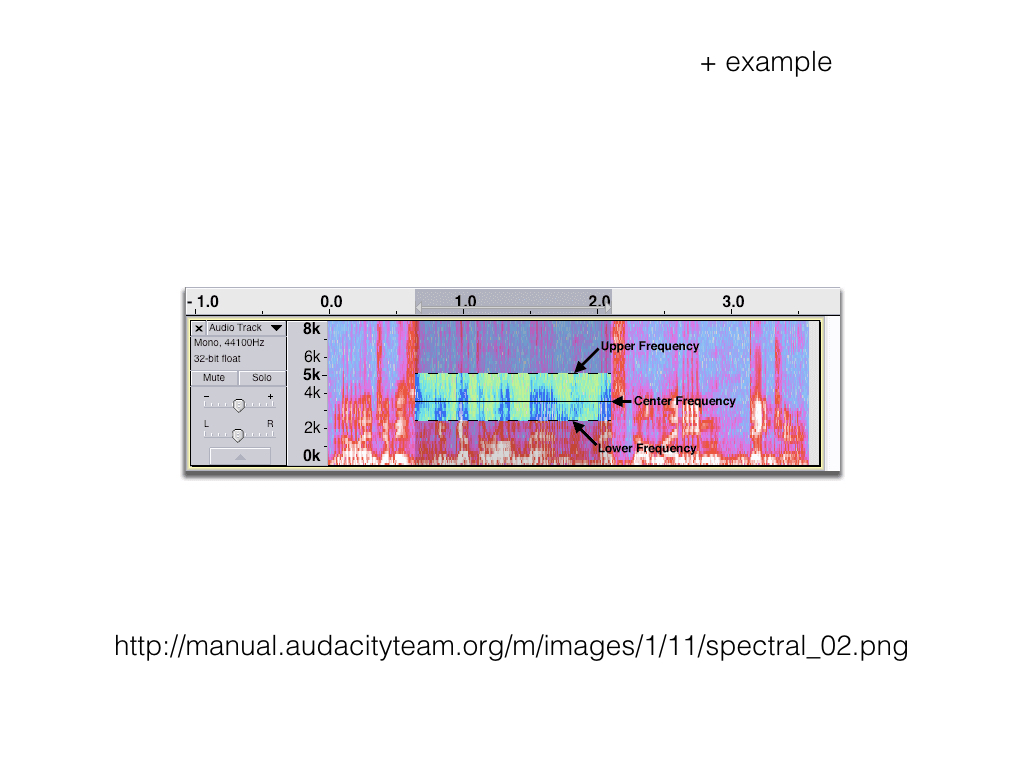Page Card

Complex Waves

Belongs to subject Complex Waves

The sine wave is the simplest sound in existence.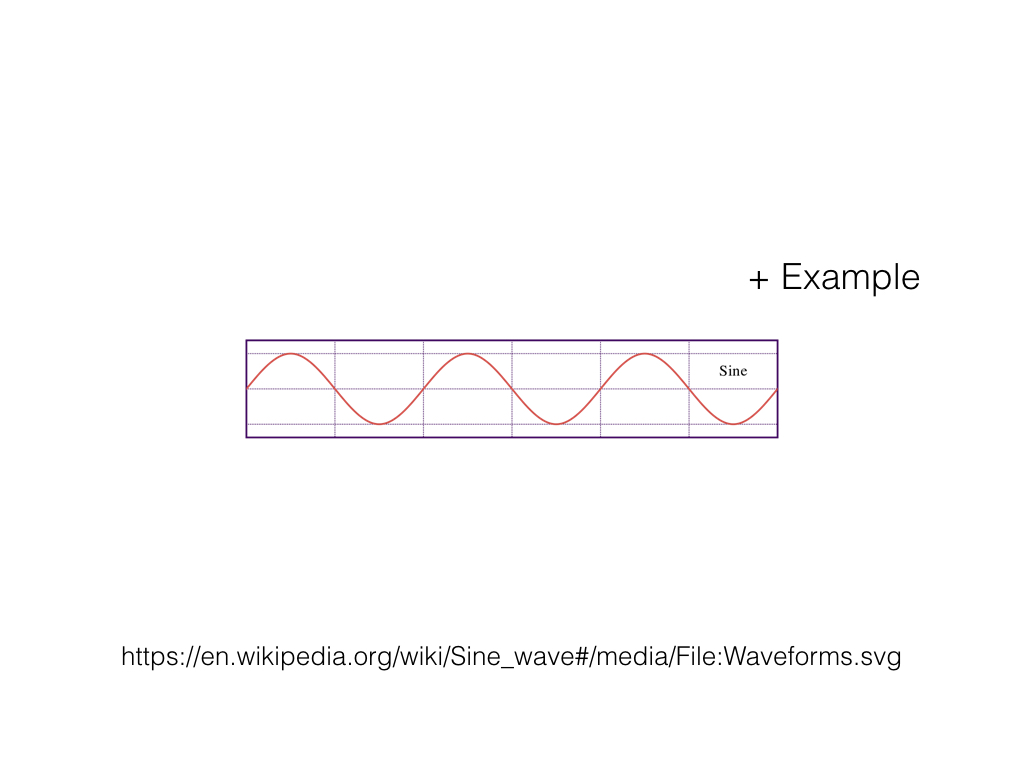The Composition of Sound

In fact, all other sound is simply a combination of multiple sine waves. This idea is known as the Fourier theorem. If we look at the triangle wave, we can see the sine waves make up the triangle wave.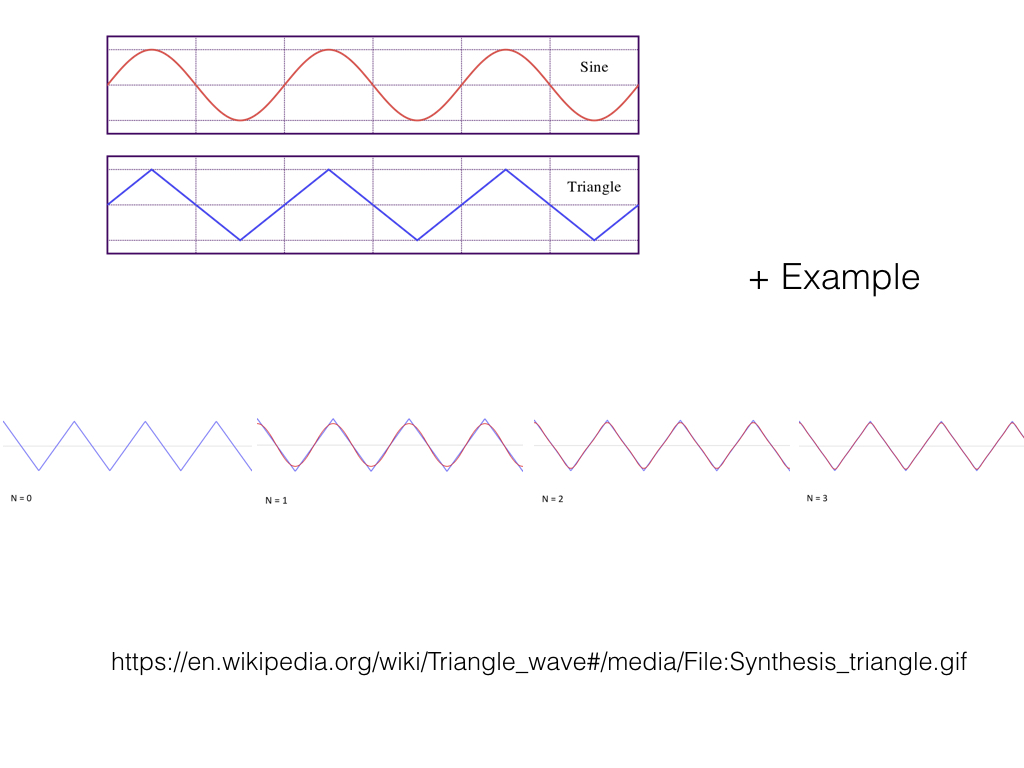The most prominent or focal sine wave is known as the fundamental frequency. The fundamental frequency is the pitch we perceive the sound at.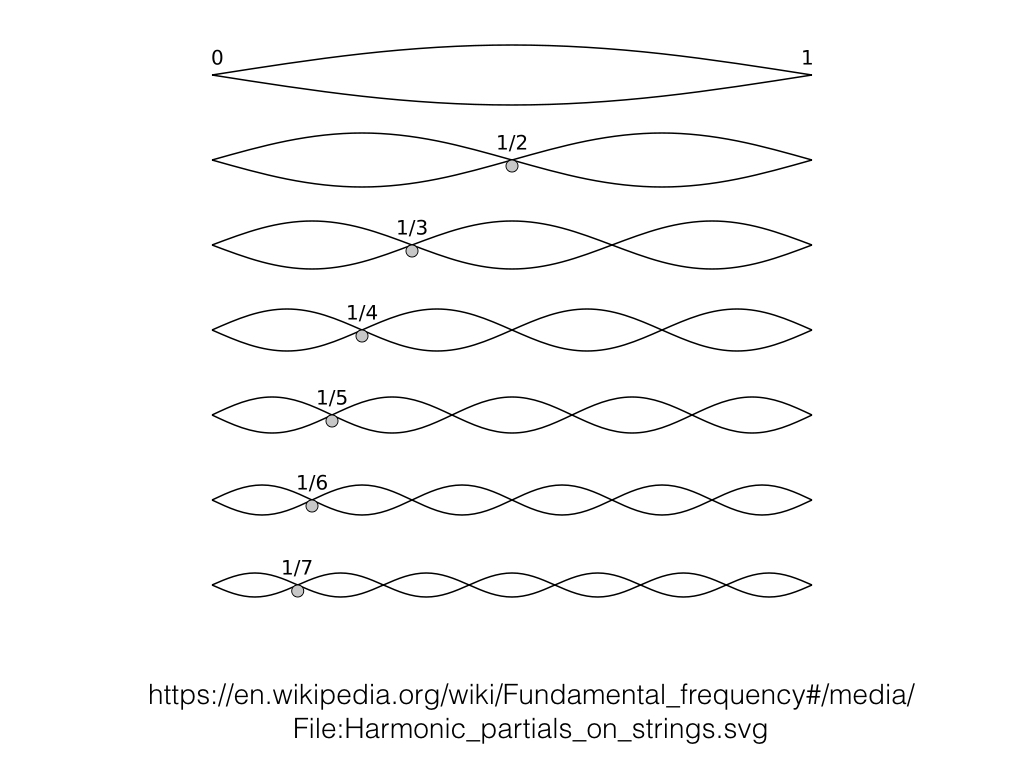The other frequencies are what produce the color of the sound. The combination of many sine waves with different frequencies, amplitudes, and phases, all of which change over time, is the spectrum, or what gives the sound color.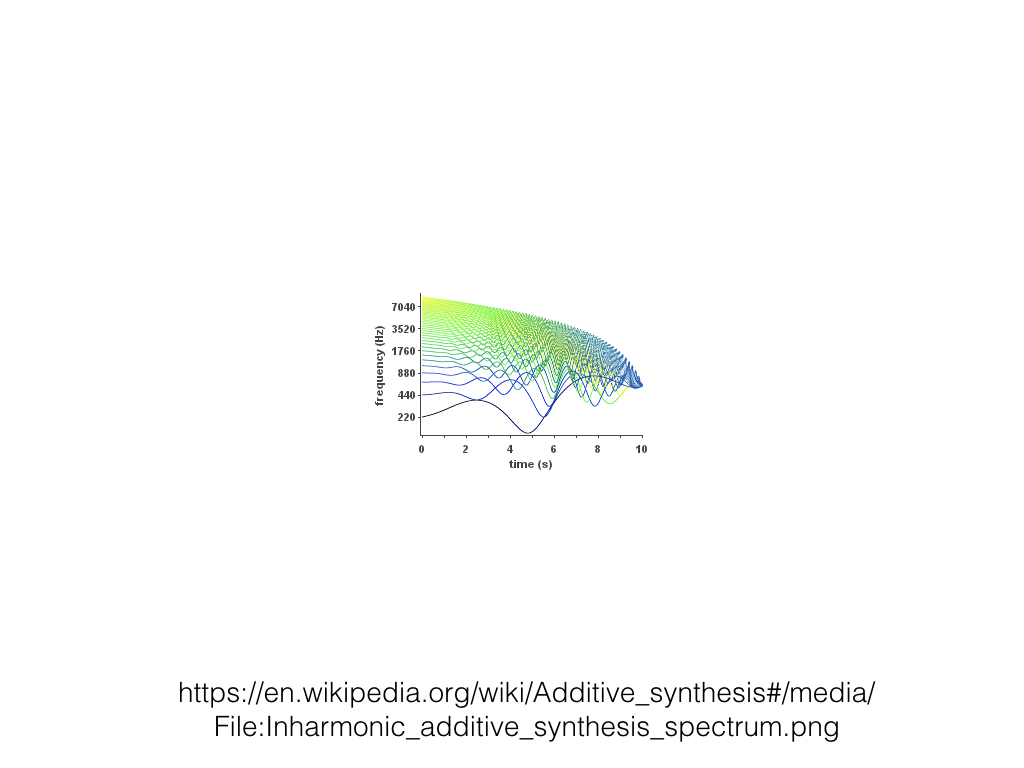Harmonics and Inharmonics

When a sine wave is an integer multiple of the fundamental frequency, we call the wave harmonic. In our triangle wave, all of the frequencies are harmonic.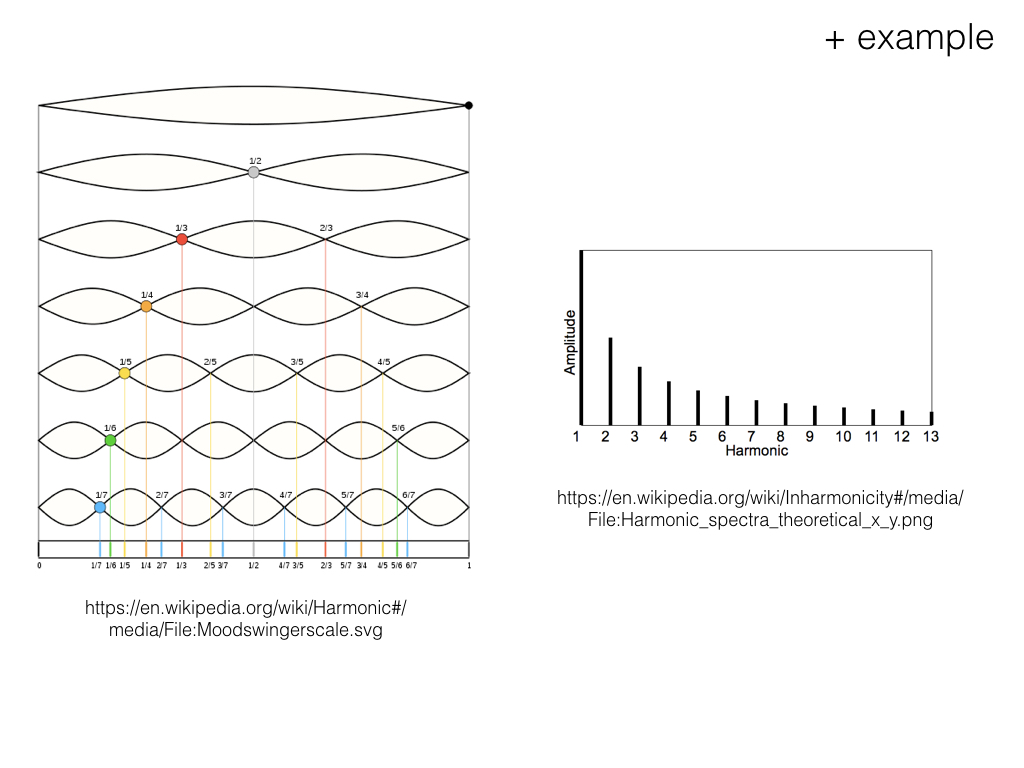When a sine wave is not an integer multiple of the fundamental frequency, we call the wave inharmonic. Inharmonic frequencies often give sounds unique colors.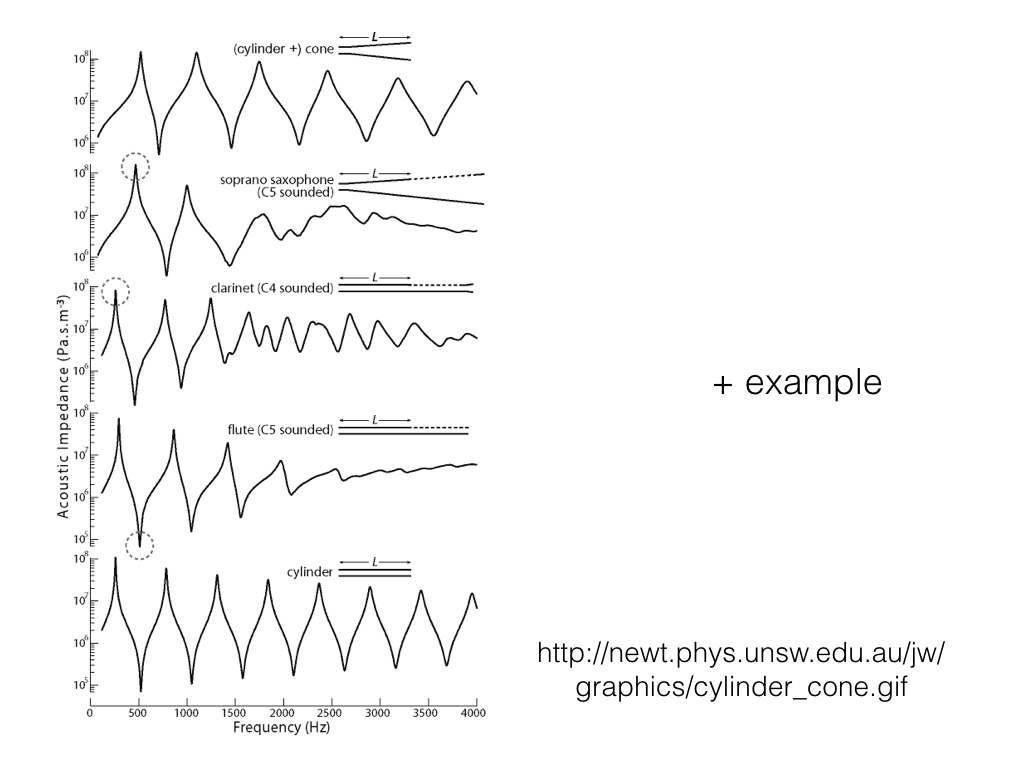When many inharmonic waves surround a single area, we call this area a formant. Importantly, we use the formants to understand speech and emotion.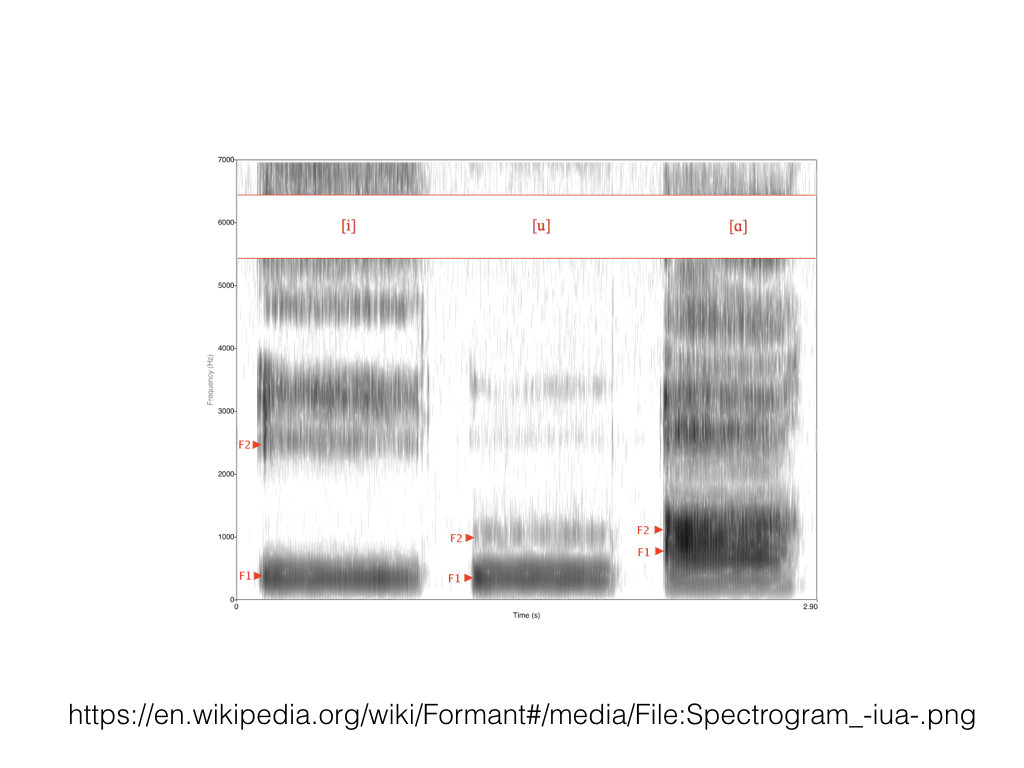We can directly change the individual sine waves within a complex wave. This technique is known as spectral editing.